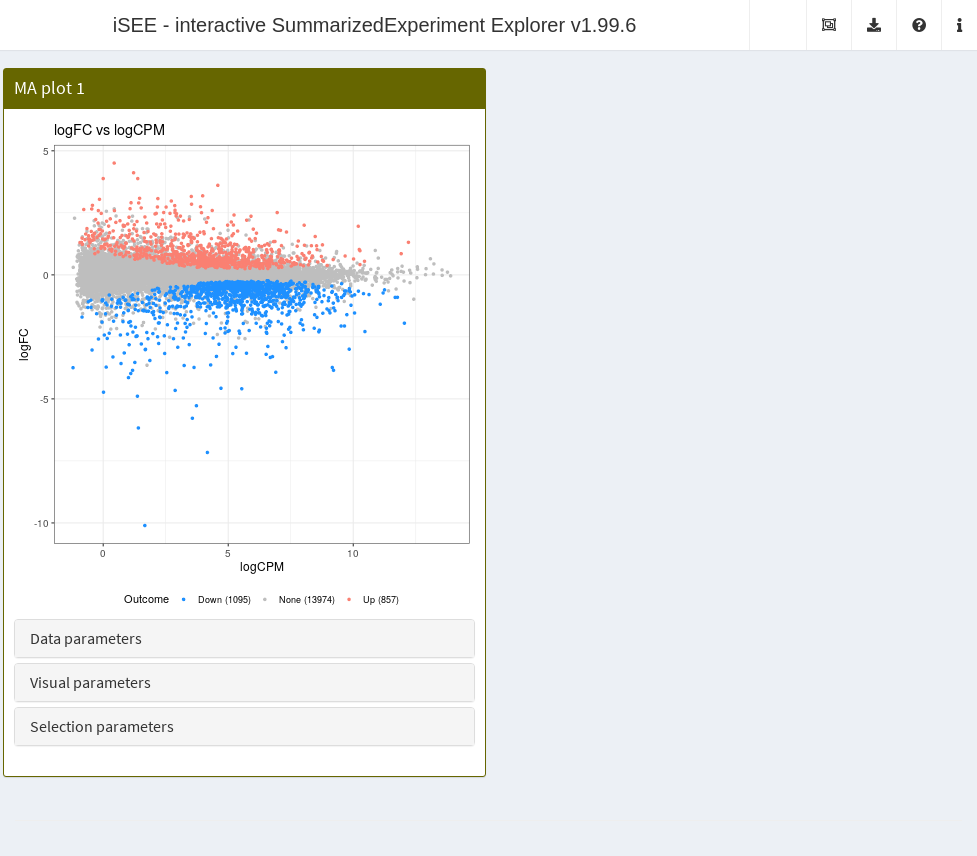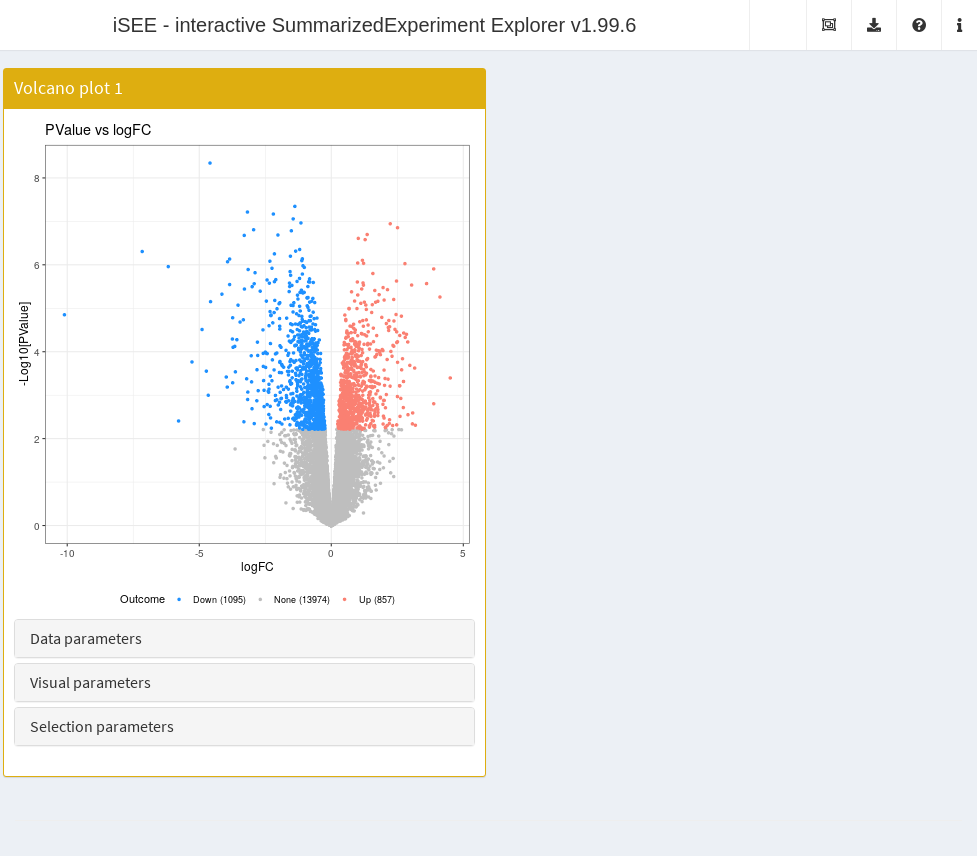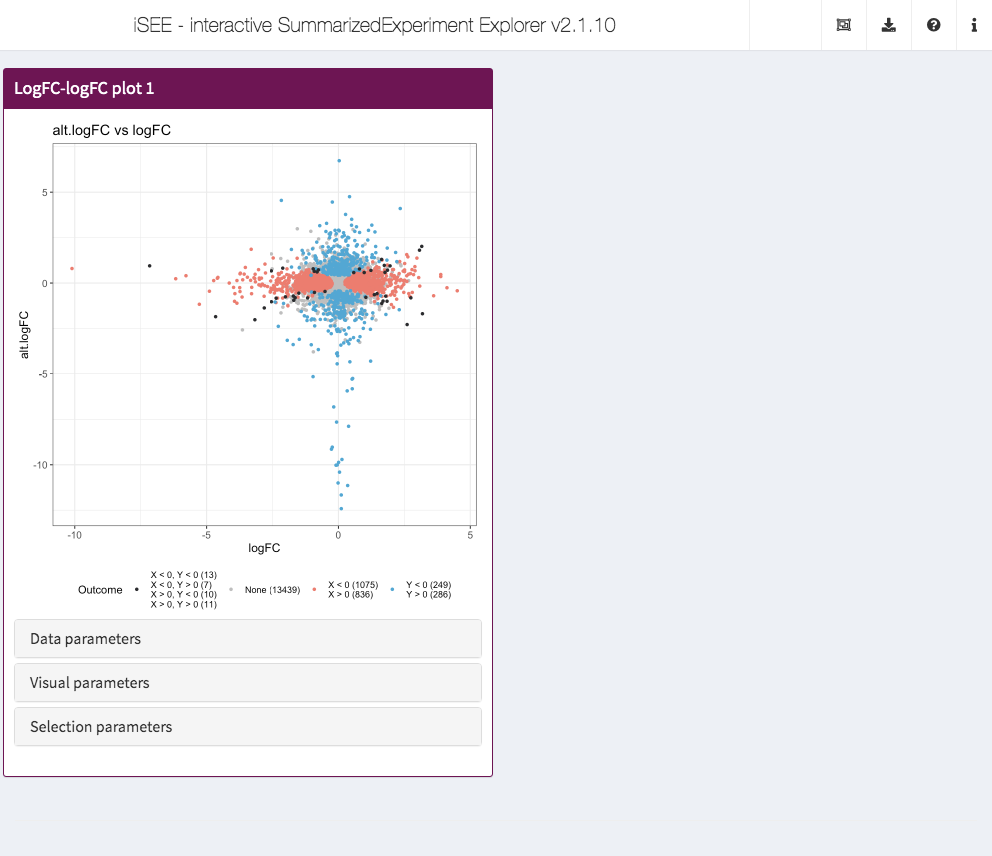# 1 Overview

The iSEE package (Rue-Albrecht et al. 2018) provides a general and flexible framework for interactively exploring `SummarizedExperiment` objects. However, in many cases, more specialized panels are required for effective visualization of specific data types. The iSEEu package implements a collection of such dedicated panel classes that work directly in the `iSEE` application and can smoothly interact with other panels. This allows users to quickly parametrize bespoke apps for their data to address scientific questions of interest. We first load in the package:

``library(iSEEu)``

All the panels described in this document can be deployed by simply passing them into the `iSEE()` function via the `initial=` argument, as shown in the following examples.

# 2 Differential expression plots

To demonstrate the use of these panels, we will perform a differential expression analysis on the airway dataset with the edgeR package. We store the resulting statistics in the `rowData` of the `SummarizedExperiment` so that it can be accessed by `iSEE` panels.

``````library(airway)
data(airway)

library(edgeR)
y <- DGEList(assay(airway), samples=colData(airway))
y <- y[filterByExpr(y, group=y\$samples\$dex),]
y <- calcNormFactors(y)

design <- model.matrix(~dex, y\$samples)
y <- estimateDisp(y, design)
fit <- glmQLFit(y, design)
res <- glmQLFTest(fit, coef=2)

tab <- topTags(res, n=Inf)\$table
rowData(airway) <- cbind(rowData(airway), tab[rownames(airway),])``````

The `MAPlot` class creates a MA plot, i.e., with the log-fold change on the y-axis and the average expression on the x-axis. Features with significant differences in each direction are highlighted and counted on the legend. Users can vary the significance threshold and apply ad hoc filters on the log-fold change. This is a subclass of the `RowDataPlot` so points can be transmitted to other panels as multiple row selections. Instances of this class are created like:

``````ma.panel <- MAPlot(PanelWidth=6L)
app <- iSEE(airway, initial=list(ma.panel))``````The `VolcanoPlot` class creates a volcano plot with the log-fold change on the x-axis and the negative log-p-value on the y-axis. Features with significant differences in each direction are highlighted and counted on the legend. Users can vary the significance threshold and apply ad hoc filters on the log-fold change. This is a subclass of the `RowDataPlot` so points can be transmitted to other panels as multiple row selections. Instances of this class are created like:

``````vol.panel <- VolcanoPlot(PanelWidth=6L)
app <- iSEE(airway, initial=list(vol.panel))``````The `LogFCLogFCPlot` class creates a scatter plot of two log-fold changes from different DE comparisons. This allows us to compare DE results on the same dataset - or even from different datasets, as long as the row names are shared. Users can vary the significant threshold used to identify DE genes in either or both comparisons. This is a subclass of the `RowDataPlot` so points can be transmitted to other panels as multiple row selections. Instances of this class are created like:

``````# Creating another comparison, this time by blocking on the cell line
design.alt <- model.matrix(~cell + dex, y\$samples)
y.alt <- estimateDisp(y, design.alt)
fit.alt <- glmQLFit(y.alt, design.alt)
res.alt <- glmQLFTest(fit.alt, coef=2)

tab.alt <- topTags(res.alt, n=Inf)\$table
rowData(airway) <- cbind(rowData(airway), alt=tab.alt[rownames(airway),])

lfc.panel <- LogFCLogFCPlot(PanelWidth=6L, YAxis="alt.logFC",
YPValueField="alt.PValue")
app <- iSEE(airway, initial=list(lfc.panel))``````# 3 Dynamically recalculated panels

To demonstrate, we will perform a quick analysis of a small dataset from the scRNAseq package. This involves computing normalized expression values and low-dimensional results using the scater package.

``````library(scRNAseq)
sce <- ReprocessedAllenData(assays="tophat_counts")

library(scater)
sce <- logNormCounts(sce, exprs_values="tophat_counts")
sce <- runPCA(sce, ncomponents=4)
sce <- runTSNE(sce)``````

The `DynamicReducedDimensionPlot` class creates a scatter plot with a dimensionality reduction result, namely principal components analysis (PCA), \(t\)-stochastic neighbor embedding (\(t\)-SNE) or uniform manifold and approximate projection (UMAP). It does so dynamically on the subset of points that are selected in a transmitting panel, allowing users to focus on finer structure when dealing with a heterogeneous population. Calculations are performed using relevant functions from the scater package.

``````# Receives a selection from a reduced dimension plot.
dyn.panel <- DynamicReducedDimensionPlot(Type="UMAP", Assay="logcounts",
ColumnSelectionSource="ReducedDimensionPlot1", PanelWidth=6L)

# NOTE: users do not have to manually create this, just
# copy it from the "Panel Settings" of an already open app.
red.panel <- ReducedDimensionPlot(PanelId=1L, PanelWidth=6L,
BrushData = list(
xmin = -45.943, xmax = -15.399, ymin = -58.560,
ymax = 49.701, coords_css = list(xmin = 51.009,
xmax = 165.009, ymin = 39.009,
ymax = 422.009), coords_img = list(xmin = 66.313,
xmax = 214.514, ymin = 50.712,
ymax = 548.612), img_css_ratio = list(x = 1.300,
y = 1.299), mapping = list(x = "X", y = "Y"),
domain = list(left = -49.101, right = 57.228,
bottom = -70.389, top = 53.519),
range = list(left = 50.986, right = 566.922,
bottom = 603.013, top = 33.155),
log = list(x = NULL, y = NULL), direction = "xy",
brushId = "ReducedDimensionPlot1_Brush",
outputId = "ReducedDimensionPlot1"
)
)

app <- iSEE(sce, initial=list(red.panel, dyn.panel))``````